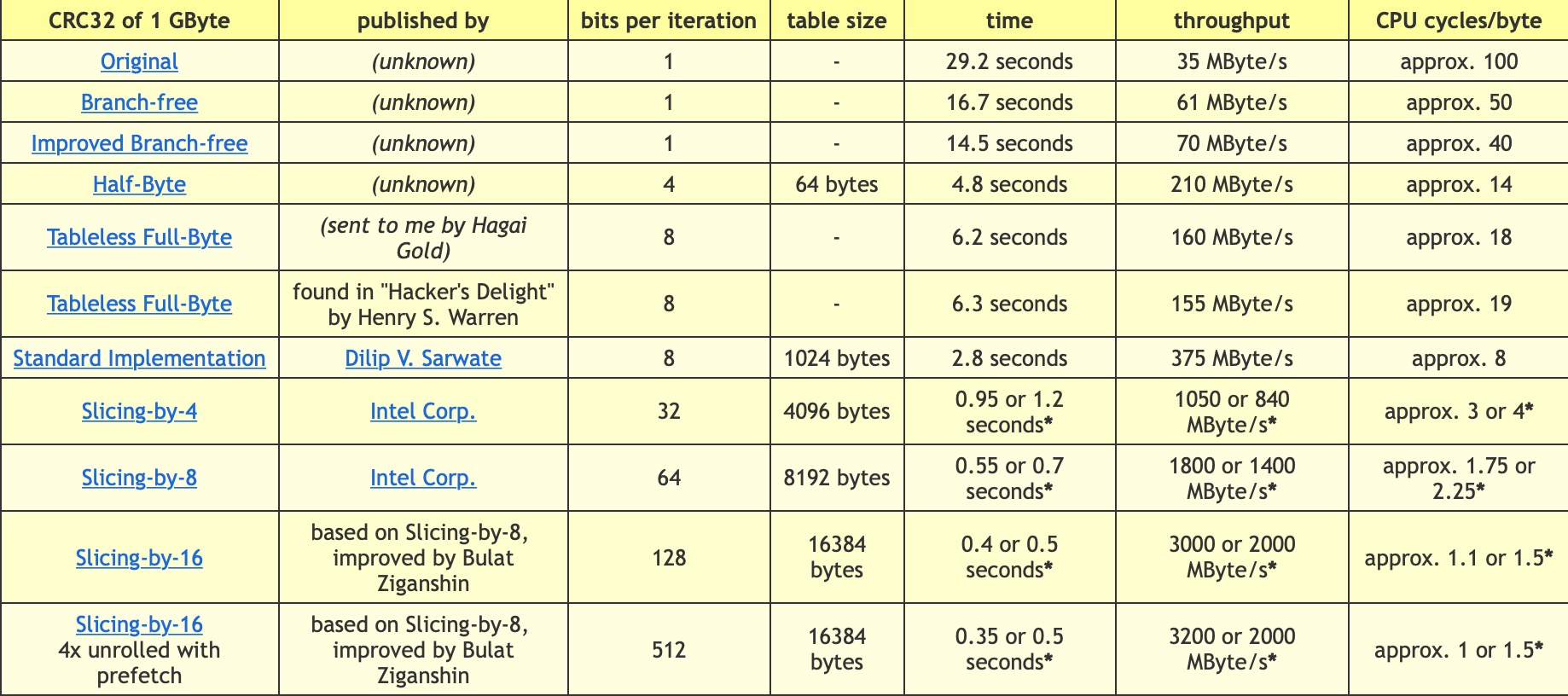2019/04/20

# CRC 算法# 优化变种算法

• 将原始查询表转化为 8 重表格
// table 是原始查询表， table[1...7] 是生成的8重表格
for (n = 0; n < 256; n++) {
crc = table[n];

for (k = 1; k < 8; k++) {
crc = table[crc & 0xff] ^ (crc >> 8);
table[k][n] = crc;
}
}

• 优化 CRC 算法，每个循环计算 8 个字节
while (len >= 8) {
crc ^= *(uint64_t *)byte; // 小端计算方式
crc = table[crc & 0xff] ^
table[(crc >> 8) & 0xff] ^
table[(crc >> 16) & 0xff] ^
table[(crc >> 24) & 0xff] ^
table[(crc >> 32) & 0xff] ^
table[(crc >> 40) & 0xff] ^
table[(crc >> 48) & 0xff] ^
table[crc >> 56];
byte += 8;
len -= 8;
}
while (len) {
crc = table[(crc ^ *byte++) & 0xff] ^ (crc >> 8);
len--;


# 实现

github.com/lrita/crc64采用上述方法，优化了redis使用的 CRC64 变种算法，使其速度从381.29 MB/s提升到1474.16 MB/s.

# PYCRC

pycrc2可以生成不同变种 CRC 算法的优化 C 代码，变种的核心参数可以通过参数--width --poly --reflect-in --xor-in --reflect-out --xor-out进行相应调整。支持的优化算法有bit-by-bit, bbb, bit-by-bit-fast, bbf, table-driven, tbl等。例如：

./pycrc.py --generate=c --algorithm=table-driven --model=crc-32 --slice-by=4